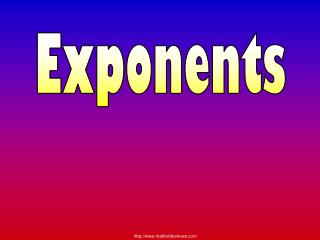DownloadDownload PresentationExponents

# Exponents

Download Presentation## Exponents

- - - - - - - - - - - - - - - - - - - - - - - - - - - E N D - - - - - - - - - - - - - - - - - - - - - - - - - - -
##### Presentation Transcript

1. Exponents

2. Exponents For Learning to Happen! • Remove all other thoughts from your mind. • Pay close attention to this lesson. • Try all of the examples. • Ignore all other distractions.

3. Exponents • An exponent is a little number high and to the right of a regular or base number. 3 8 Exponent Base

4. Exponents This exponent is read 8 to the 3rd power, or 8 cubed. 3 8 Exponent Base

5. Exponents An exponent tells how many times a number is multiplied by itself. 3 8 Exponent Base

6. Exponents This exponent means 8•8•8 = 512 3 8 Exponent Base

7. Exponents • This exponent is read • six to the fourth power. Exponent 6 4 Base

8. Exponents • This exponent is read three to the 2nd power or three squared. Exponent 3 2 Base

9. Exponents Read These Exponents 2 7 7 to the 2nd power or 7 squared

10. Exponents Read These Exponents 3 10 10 to the 3rd power or 10 cubed

11. Exponents What is the Exponent? 3 2 •2 • 2 = 2

12. Exponents What is the Exponent? 4 a a •a • a • a=

13. Exponents What is the Exponent? 3 (bc)(bc)(bc) = (bc) NOTICE that you keep the parenthesis around the variables (bc) because you are cubing all the (bc)

14. Exponents Write the Verbal Phase Power Verbal Phrase Means “Seven to the 2nd power” “Seven squared” “Five to the 3rd power” “Five cubed”

15. Exponents Write the Verbal Phase Power Verbal Phrase Meaning “Nine to the 4th power” “Two to the 5th power”

16. Exponents How to Multiply Out Exponents = 3 •3 • 3 •3 4 3 9 27 81

17. Exponents How to Multiply Out Exponents = 2 •2 • 2 •2 • 2 5 2 4 8 16 32

18. #1 Exponents • 3 • 8 • Exponent • An exponent tells how many times a number is • multiplied by itself. • 8•8•8 • = 512 • Base • Factored Form • Exponential Form • 3 • 3 • a • a • a • 32a3 • x • y • x • 2 • 2x2y1

19. #3 1) 3) 2) 4) Evaluate The Power • To find 54 on my calculator I type in 5 ^ 4 = 625 5 4 = 625 xy 5 4 5 = 625 x 4 = 625 yx Circle the method that works on your calculator!

20. “Evaluate” mean to solve! 1) 3) 2) 4) Exponents Evaluate the Power

21. Exponents Addition: -One-Dimensional Growth “Three added to four” = + 3 4 7 units = + Multiplication: Two-Dimensional Growth “Three times four” or “Three groups of four” + + = 3(4) 4 4 4 12 square units = = + +

22. Exponents Exponents: Three-Dimensional Growth “Three to the third power” “Three to the fourth power” 3 3 3

23. Perimeter Area Volume Exponents 5 5 5 5 5 5 5 5 5 P = s+s+s+s P = 4s “Five Squared” “Five Cubed” One Dimensional Two Dimensional Three Dimensional

24. Exponents The End!!! Check the last slide to make sure your notes are complete

25. #1 Exponents & Powers • An exponent or power tells how many • times a number is multiplied by itself. 4 Exponent 3 Base “Five to the 2nd power” “Five squared” “Seven to the 3rd power” “Seven cubed”

26. #2 1) 3) 2) 4) Evaluate The Power